# Math in Focus Grade 1 Chapter 4 Practice 5 Answer Key Making Fact Families

This handy Math in Focus Grade 1 Workbook Answer Key Chapter 4 Practice 5 Making Fact Families detailed solutions for the textbook questions.

## Math in Focus Grade 1 Chapter 4 Practice 5 Answer Key Making Fact Families

Write a fact family for each picture.

Example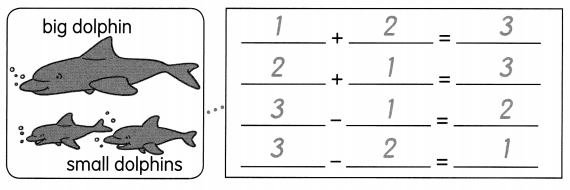Question 1.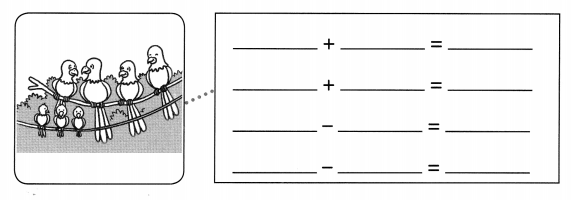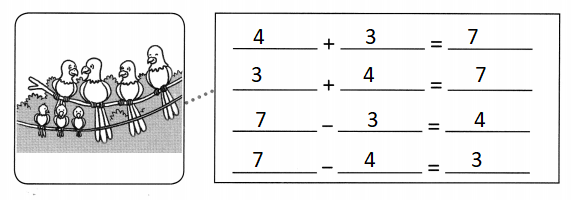Explanation:
An addition fact is the sum of two specific addends that will always equal the same result.
A subtraction fact family is a group of related math facts that use the same three numbers. Subtraction fact families also include addition, which is the inverse or opposite operation of subtraction.

Question 2.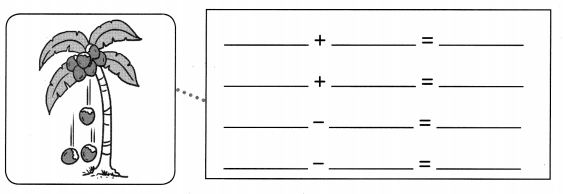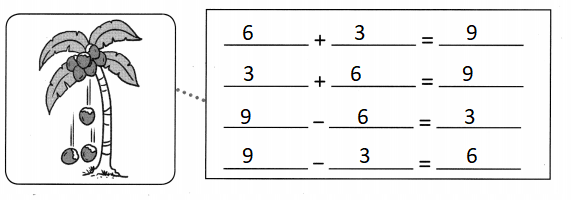Explanation:
An addition fact is the sum of two specific addends that will always equal the same result.
A subtraction fact family is a group of related math facts that use the same three numbers. Subtraction fact families also include addition, which is the inverse or opposite operation of subtraction.

Solve.

Question 3.
Simone has some tomatoes. She throws away 5 rotten tomatoes. She has 4 tomatoes left. How many tomatoes did she have at first?
___ – 5 = 4
5 + 4 = __ is the related addition fact.
She had _______ tomatoes at first.
9  – 5 = 4
5 + 4 = 9 is the related addition fact.
She had 9 tomatoes at first.
Explanation:
An addition fact is the sum of two specific addends that will always equal the same result.
A subtraction fact family is a group of related math facts that use the same three numbers. Subtraction fact families also include addition, which is the inverse or opposite operation of subtraction.

Question 4.
Marcus has 6 magnets. Susan gives him some magnets. Marcus now has 9 magnets. How many magnets did Susan give Marcus?
6 + __ = 9
9 – 6 = ___ is the related subtraction fact.
Susan gave Marcus ___ magnets.
6 + 3 = 9
9 – 6 = 3 is the related subtraction fact.
Susan gave Marcus 3 magnets.
Explanation:
An addition fact is the sum of two specific addends that will always equal the same result.
A subtraction fact family is a group of related math facts that use the same three numbers. Subtraction fact families also include addition, which is the inverse or opposite operation of subtraction.

Find the missing number.

Question 5.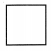+ 5 = 10
5
Explanation:
5 + 5 = 10
10 – 5 = 5
Used the subtraction fact to find the additional fact

Question 6.
2 += 7
5
Explanation:
7 – 2 = 5
Used the subtraction fact to find the additional fact

Question 7.– 8 = 2
9 –= 3